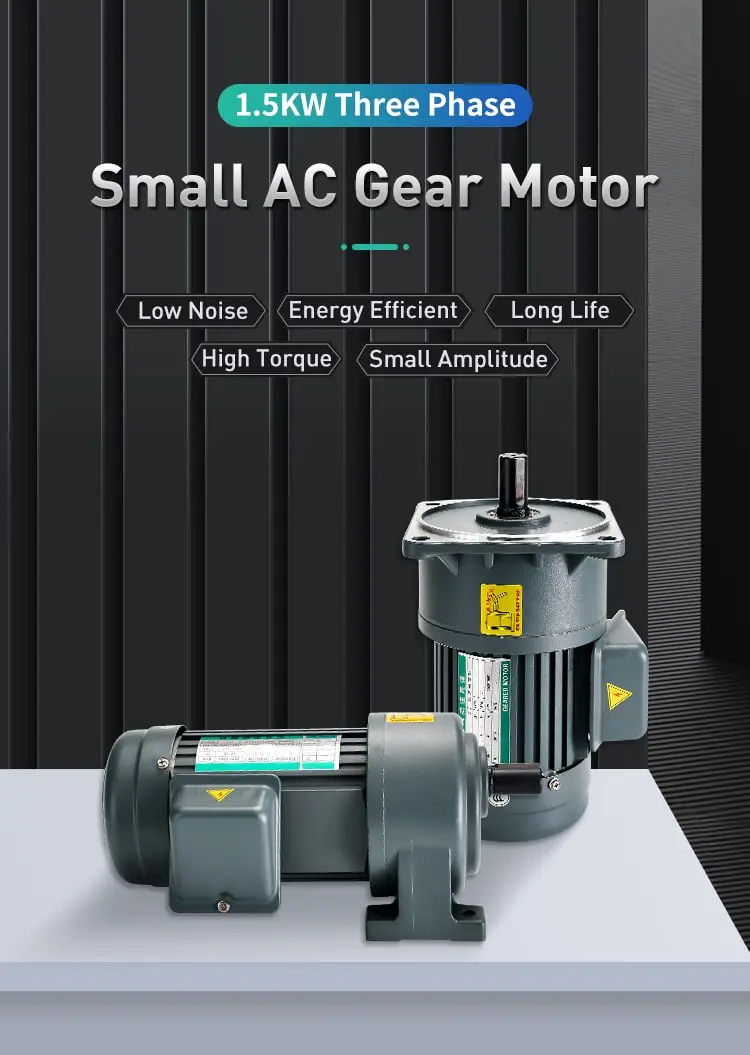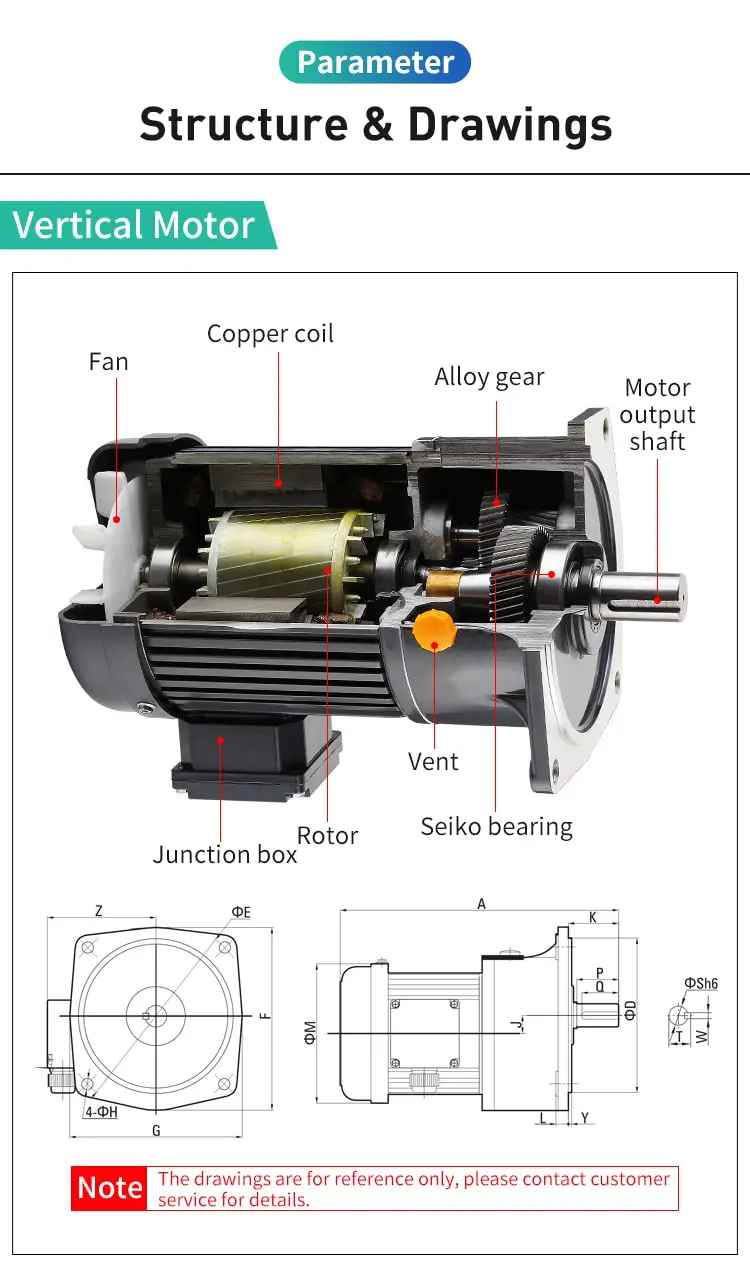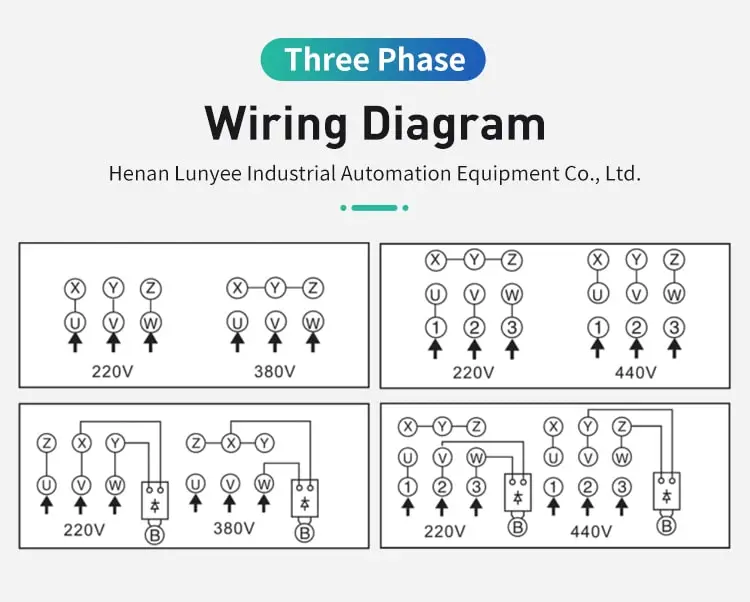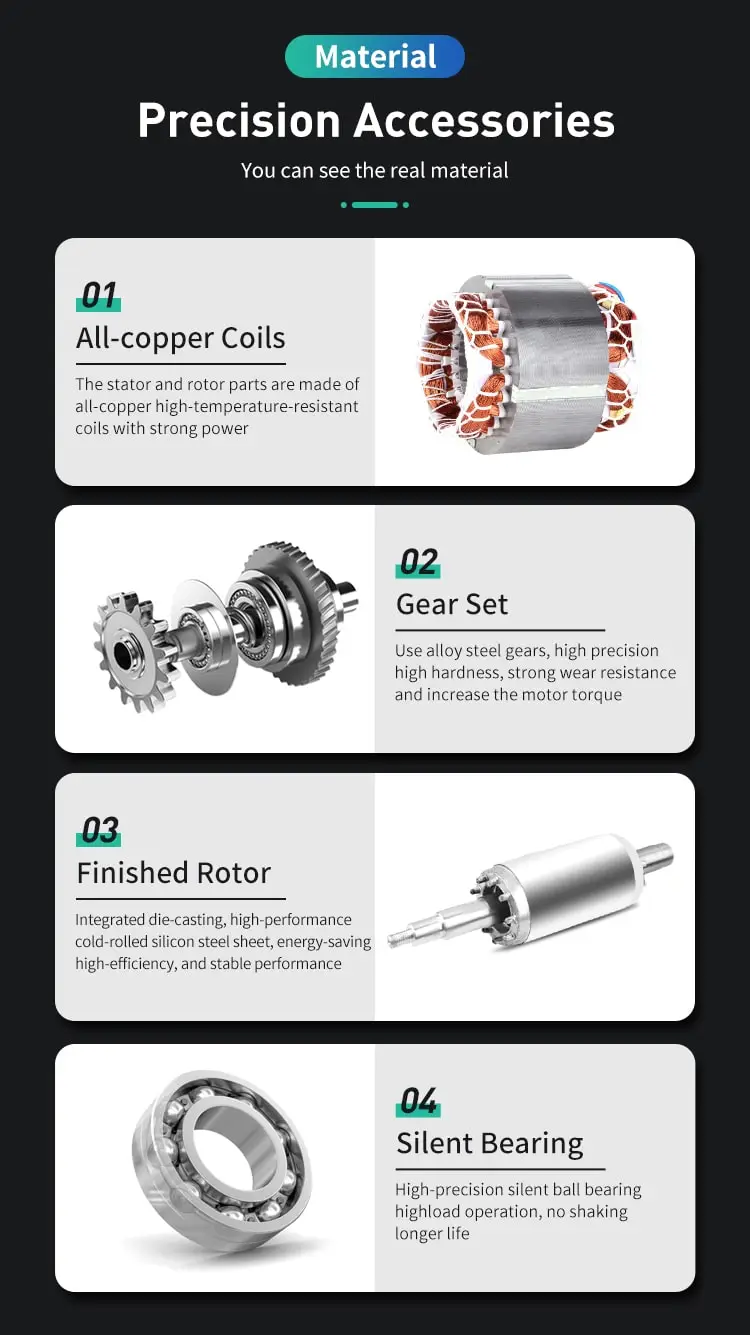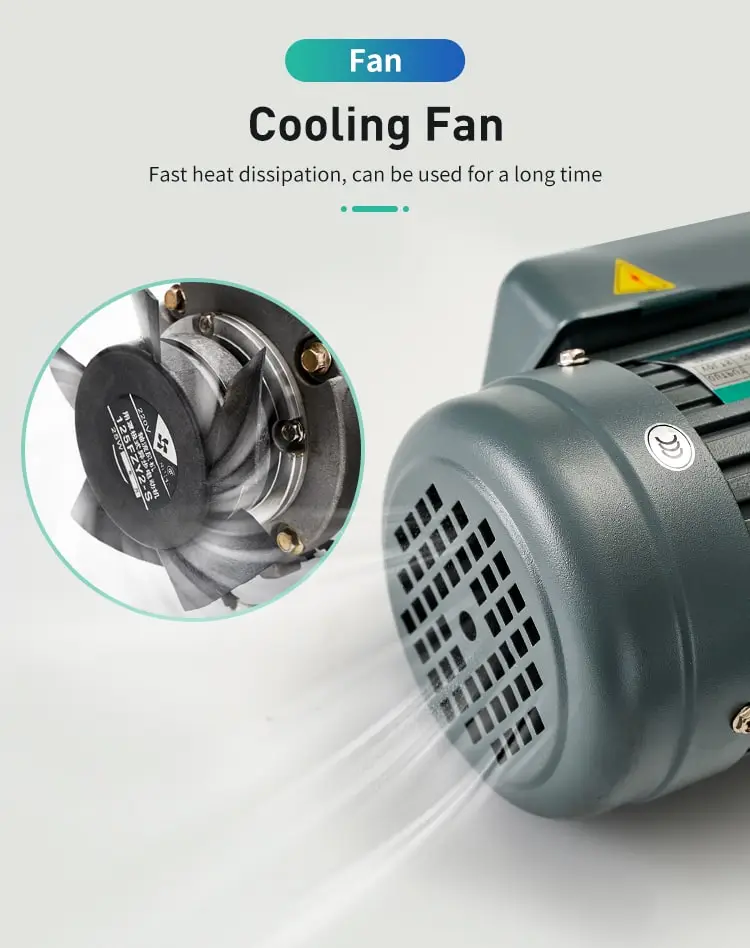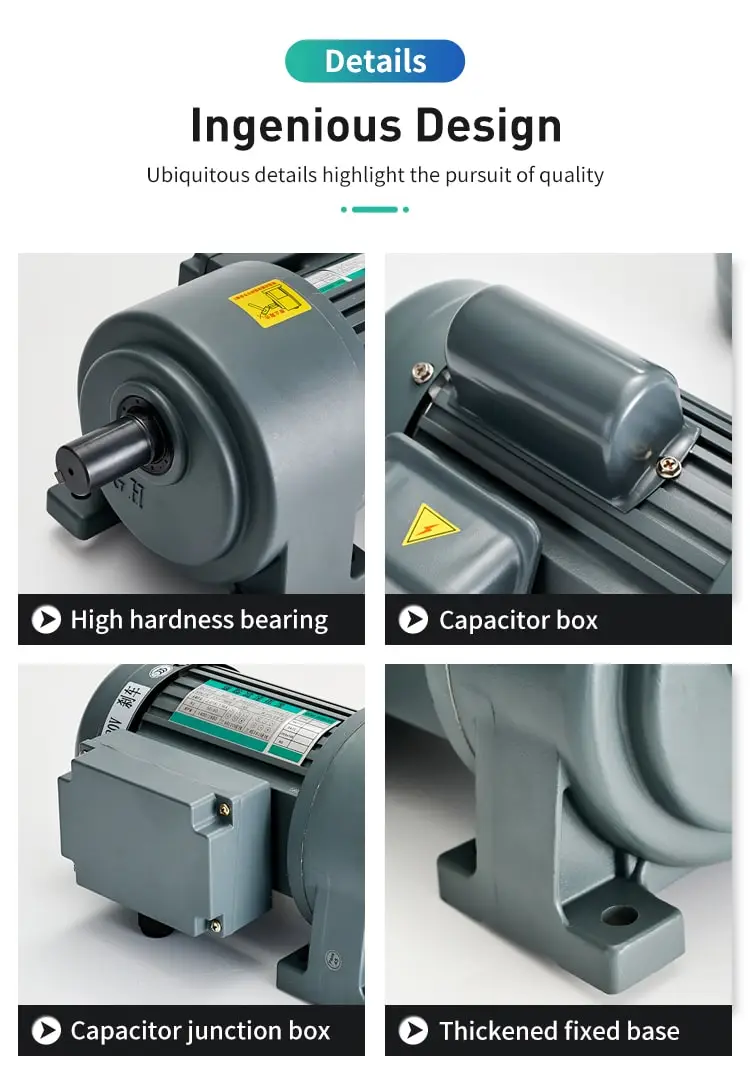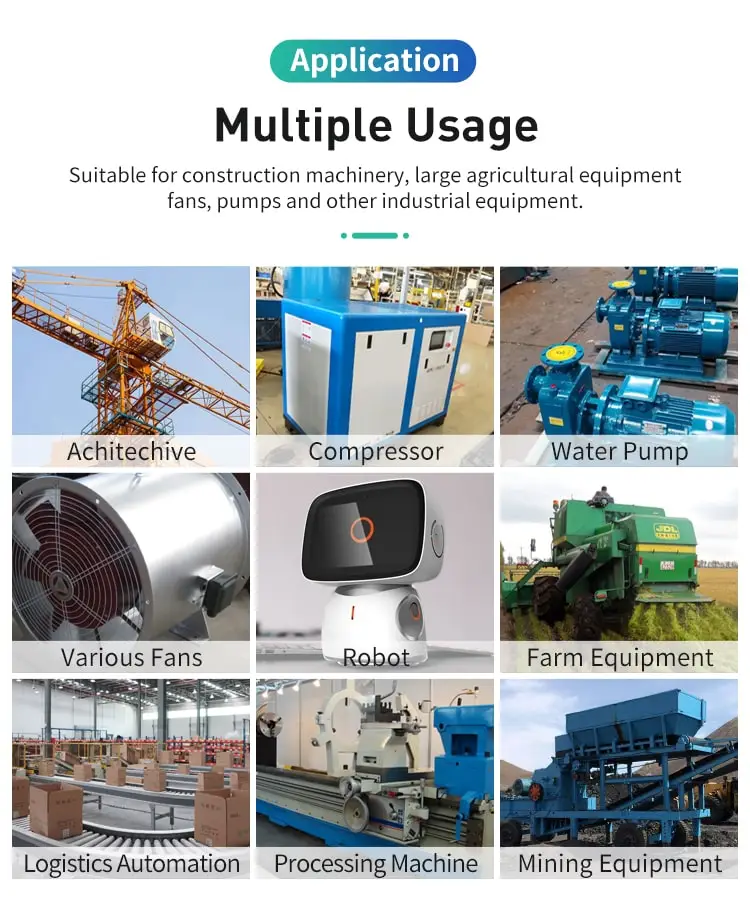By Jasmine 2023-03-21 14:47:50

#### How to change the speed of an ac induction motor?

The speed of the induction motor can be changed by varying the frequency, number of poles or slip. By changing the stator poles, the speed of the induction motor can be changed. The number of stator poles can be changed by, Multiple Stator Windings.

There are several methods for changing the speed of an AC induction motor, including the following:

1. Changing the frequency of the AC power supply: The speed of an AC induction motor is directly proportional to the frequency of the AC power supply. By changing the frequency of the AC power supply, the speed of the motor can be changed. For example, increasing the frequency of the AC power supply will increase the speed of the motor, and decreasing the frequency of the AC power supply will decrease the speed of the motor.

2. Using a variable frequency drive (VFD): A variable frequency drive (VFD) is an electronic device that is used to control the speed of an AC induction motor. The VFD receives a DC input voltage and converts it into a variable frequency AC output voltage. By controlling the frequency of the AC output voltage, the VFD can control the speed of the motor.

3. Using a motor with a wound rotor: An AC induction motor with a wound rotor has a rotor that is composed of a set of windings that are connected to a slip ring. By applying a variable resistance to the rotor windings, the speed of the motor can be changed. This method is commonly used in fans and blowers, where the speed of the motor needs to be changed to adjust the flow rate of the air.

4. Using a motor with a cage rotor: An AC induction motor with a cage rotor has a rotor that is composed of a set of bars that are short-circuited by end rings. By adding external resistance to the rotor circuit, the speed of the motor can be changed. This method is commonly used in machines such as lathes, where the speed of the motor needs to be changed to adjust the cutting speed.

In summary, as a type of AC motors , there are several methods for changing the speed of an AC induction motor, including changing the frequency of the AC power supply, using a variable frequency drive (VFD), using a motor with a wound rotor, and using a motor with a cage rotor. By using one or more of these methods, the speed of an AC induction motor can be changed to meet the requirements of a particular application.